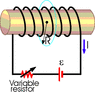# Mutual Induction of a solenoid and a coil

• shuangfei217

## Homework Statement

A single-turn circular loop of radius R = 0.235 m is coaxial with a long 1780 turn solenoid of radius 0.0435 m and length 0.850 m, as seen in the figure below. The variable resistor is changed so that the solenoid current decreases linearly from 6.12 A to 1.46 A in 0.230 s. Calculate the induced emf in the circular loop. (The field just outside the solenoid is small enough to be negligible.)

## Homework Equations

M=N*∏*μ0*n*r^2

Vind = - N*∏*μ0*n*r^2 * di/dt

di/dt=(1.46-6.12)/0.23

## The Attempt at a Solution

I used mutual inductance equ to find the potential difference induced

N is 1780, n=N/length, r=0.0435

but I still can't get the correct answer. I don't know where I did wrong here. Does anybody help me to find the answer? Thank you

#### Attachments

•serw2054.gif
6.5 KB · Views: 768
Does anyone can help me?